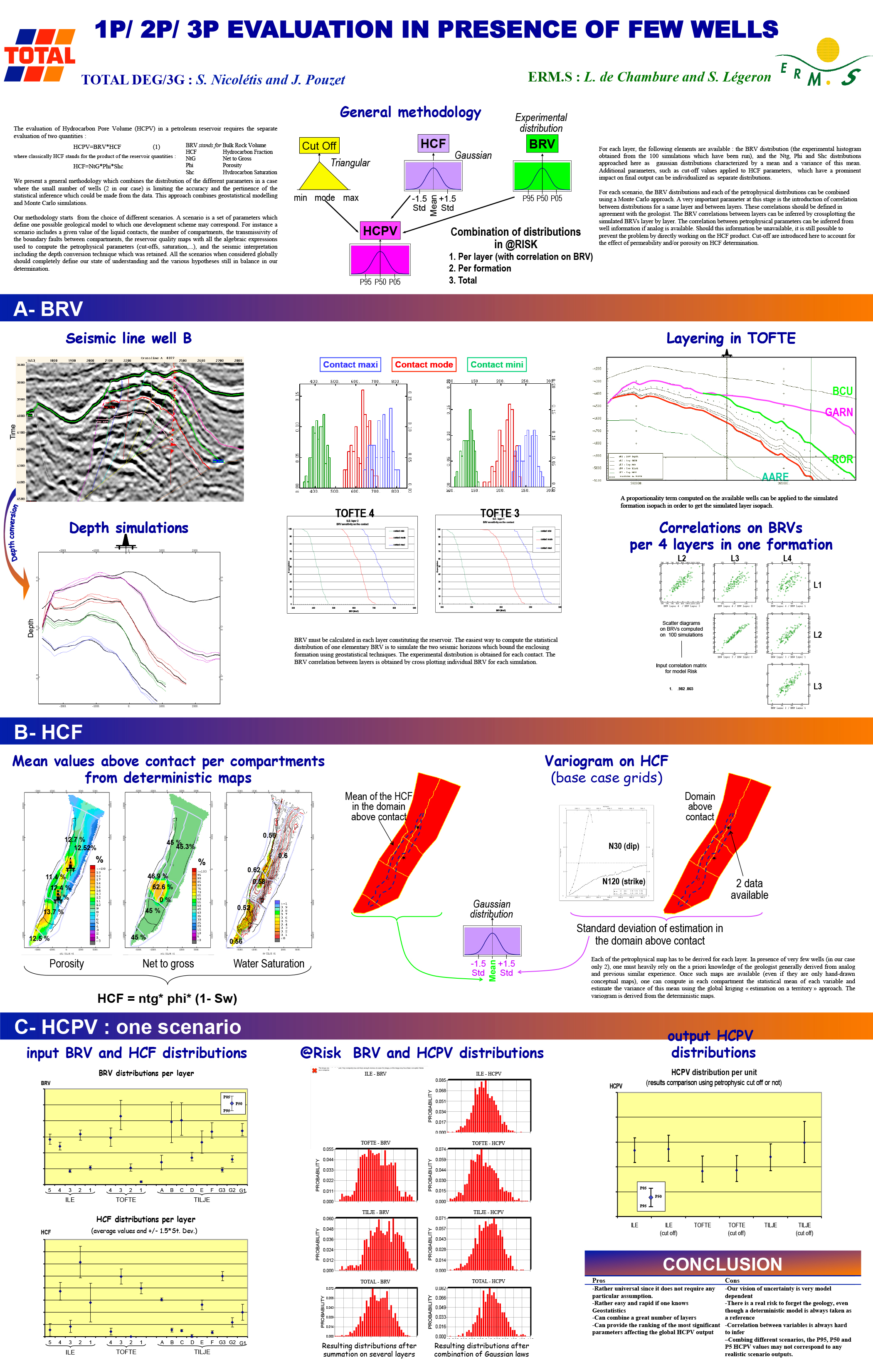The evaluation of Hydrocarbon Pore Volume (HCPV) in a petroleum reservoir requires the separate evaluation of two quantities :
HCPV=BRV*HCF (1)

where classically HCF stands for the product of the reservoir quantities :
HCF=NtG*Phi*Shc
We present a general methodology which combines the distribution of the different parameters in a case where the small number of wells (2 in our case) is limiting the accuracy and the pertinence of the statistical inference which could be made from the data. This approach combines geostatistical modelling and Monte Carlo simulations. Our methodology starts from the choice of different scenarios. A scenario is a set of parameters which define one possible geological model to which one development scheme may correspond. For instance a scenario includes a given value of the liquid contacts, the number of compartments, the transmissivity of the boundary faults between compartments, the reservoir quality maps with all the algebraic expressions used to compute the petrophysical parameters (cut-offs, saturation,…), and the seismic interpretation including the depth conversion technique which was retained. All the scenarios when considered globally should completely define our state of understanding and the various hypotheses still in balance in our determination.# software solutions